français | español | polski | 日本語 |

Pokemon MATH 67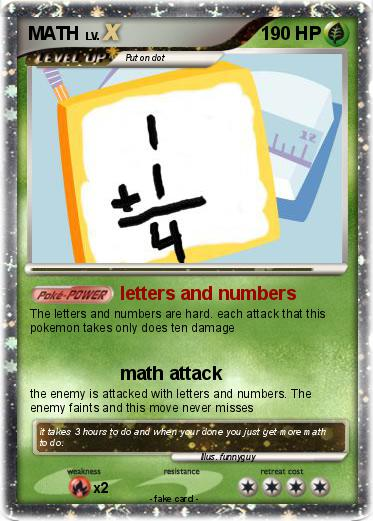Language Card : english

Creation Date : 26 July 2013

### Pokemon Passport

Name : MATH

Serie : Diamond & Pearl - Pokémon LV.X

Type : Grass

Attack 1 : letters and numbers
The letters and numbers are hard. each attack that this pokemon takes only does ten damage

Attack 2 : math attack
the enemy is attacked with letters and numbers. The enemy faints and this move never misses

Comments : it takes 3 hours to do and when your done you just get more math to do.

Illustrator : funnyguy

### Vote for this card

(4 votes)

Related cards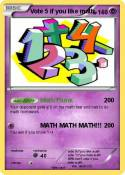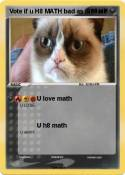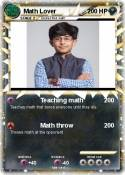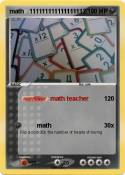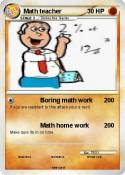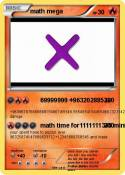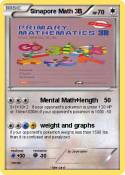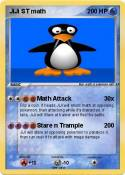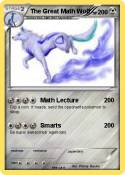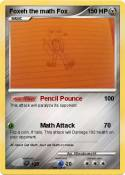Khan academy | Vote 5 if you hate math | Math Mann | I Can Do Math Everyday! | Math Teacher | MATh | math team | Math Book | Math knight | Brice | Math | Math Teacher | Math :( | math 100 x | me in math | Math man | Daniel EX | Nerd | math | Math Homework | Cool Math | math doesn't lie | Tushar | Math 24 | Math Knight | Math Man | Math | Boring Math Teacher | Math | Mrs Ferriola | 1+1 | Ratey hte math cat | St Math | Mrs Baker | Math | Math | Math | Math | more math | math | Asian guy | Asian guy | School | Math | Math Quiz | Vote if you like math | Matt's math degree | ??????????????? | njo | Math | Pi | ??? | math | PIE | can you Math????? | math pig | connor | math | M I C H A L | Homework | Mr pop | Nerdy | F-Man | = | Math | math | Bob | LEAFATARR | Kid | God Damn

Comments

Pokémon is a registered trademark of Nintendo, Creatures, Game Freak and The Pokémon Company
MyPoKeCaRd.cOm 0.9 - Propulsed by jquery, php, gd and spip - XHTML 1 and CSS2 valid page
MyPokeCard.com is a funny site to design your own pokemon card, vote for the best pokemon cards and create pokemon colorings